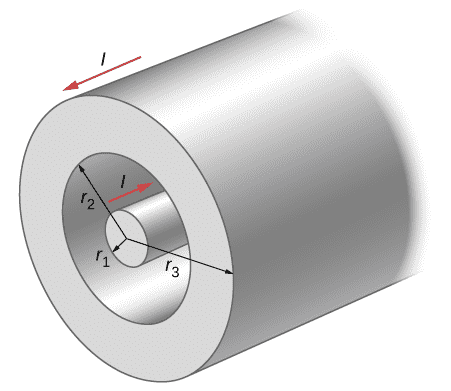# Ampere's Law with a coaxial cable

Ignitia

## Homework Statement

A portion of a long, cylindrical coaxial cable is shown
in the accompanying figure. A current I flows down the
center conductor, and this current is returned in the outer
conductor. Determine the magnetic field in the regions (a)
R ≤ r1, (b) r2 ≥ R ≥ r1, (c) r3 ≥ R ≥ r2, and (d)
R ≥ r3. Assume that the current is distributed uniformly
over the cross sections of the two parts of the cable.## Homework Equations

B∫dl = μI

Inner current = I1
Outer Current = I2

I = J*A
J = Current Density
A = Area

## The Attempt at a Solution

(a) R ≤ r1
B∫dl = μI
B*2πR = μ*J*A
B*2πR = μ*(I/πr12)*πR2
B = (μ*I*R)/2πr12
The answer given states it's only B = (μ*I*R)/r12 Why is that?

(b) r2 ≥ R ≥ r1

My thinking was that since this is outside the conductor, B = 0T since I = 0. The answer says the enclosed charge would be I, not 0, but doesn't go into any further detail. Can someone please explain why?

(c) r3 ≥ R ≥ r2
My answer was the same as a and was wrong, and likely related to B. the book stated that I = I1 - I2. Why is this?

(d) R ≥ r3
Since it's outside the conductor, then I = 0 and B = 0T This was correct.

Thanks for the help.

#### Attachments

Staff Emeritus
•